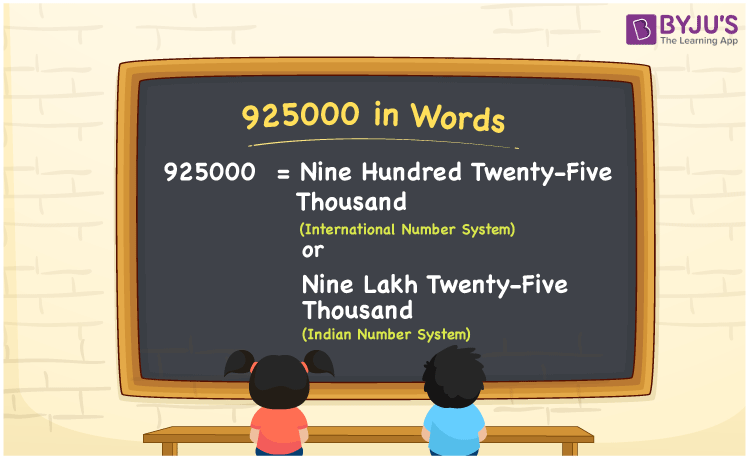# 925000 in words

925000 in words is written as Nine Lakh Twenty Five Thousand in the Indian Numeral System and Nine Hundred Twenty Five Thousand in the International Numeral system. The article on Numeral System can give you an idea about the difference between both systems. The number 925000 is used in expressions that relate to huge quantities, like money, population, area, social media views, distance between celestial bodies. If expressed in terms of money, we can say that “In an interview, an experienced candidate was offered Nine Lakh Twenty Five Thousand Rupees as the annual package.” In terms of area, we can use 925000 as “Nigeria has a total area of less than Nine Hundred Twenty Five Thousand square kilometers”.

Numeral System →

Indian Numeral System

International Numeral System

925000 in words

Nine Lakh Twenty Five Thousand

Nine Hundred Twenty Five Thousand

Nine Lakh Twenty Five Thousand in Numbers

9,25,000

Nine Hundred Twenty Five Thousand in Numbers

925,000

## 925000 in English Words## How to Write 925000 in Words?

We can convert 925000 to words using a place value chart. The number 925000 has 6 digits, so let’s make a chart that shows the place value up to 6 digits. Different tables to show both Numeral Systems are given.

Indian System

 Lakhs Ten Thousands Thousands Hundreds Tens Ones 9 2 5 0 0 0

Thus, we can write the expanded form as:

9 × Lakhs + 2 × Ten thousand + 5 × Thousand + 0 × Hundred + 0 × Ten + 0 × One

= 9 × 100000 + 2 × 10000 + 5 × 1000 + 0 × 100 + 0 × 10 + 0 × 1

= 925000

= Nine Lakh Twenty Five Thousand.

International System

 Hundred Thousands Ten Thousands Thousands Hundreds Tens Ones 9 2 5 0 0 0

Thus, we can write the expanded form as:

9 × Hundred Thousand + 2 × Ten thousand + 5 × Thousand + 0 × Hundred + 0 × Ten + 0 × One

= 9 × 100000 + 2 × 10000 + 5 × 1000 + 0 × 100 + 0 × 10 + 0 × 1

= 925000

= Nine Hundred Twenty Five Thousand.

925000 is the natural number that is succeeded by 924999 and preceded by 925001.

925000 in words – Nine Lakh Twenty Five Thousand (Indian System) and Nine Hundred Twenty Five Thousand (International System).

Is 925000 an odd number? – No.

Is 925000 an even number? – Yes.

Is 925000 a perfect square number? – No.

Is 925000 a perfect cube number? – No.

Is 925000 a prime number? – No.

Is 925000 a composite number? – Yes.

## Solved Example

1. Write the number 925000 in expanded form.

Solution: = 9 × 100000 + 2 × 10000 + 5 × 1000 + 0 × 100 + 0 × 10 + 0 × 1

We can write 925000 = 900000 + 20000 + 5000 + 0 + 0 + 0

Therefore it will be

= 9 × 100000 + 2 × 10000 + 5 × 1000 + 0 × 100 + 0 × 10 + 0 × 1